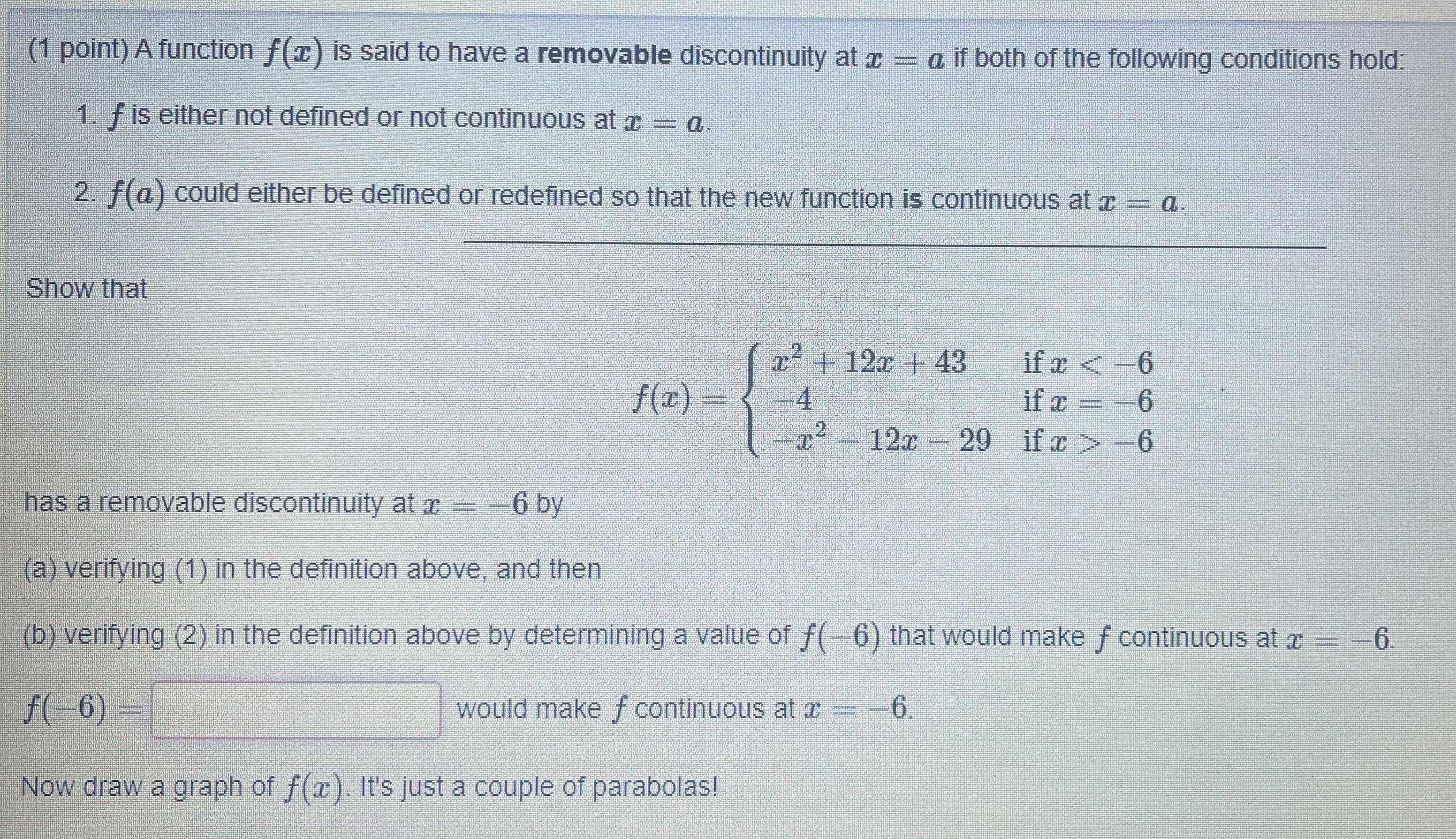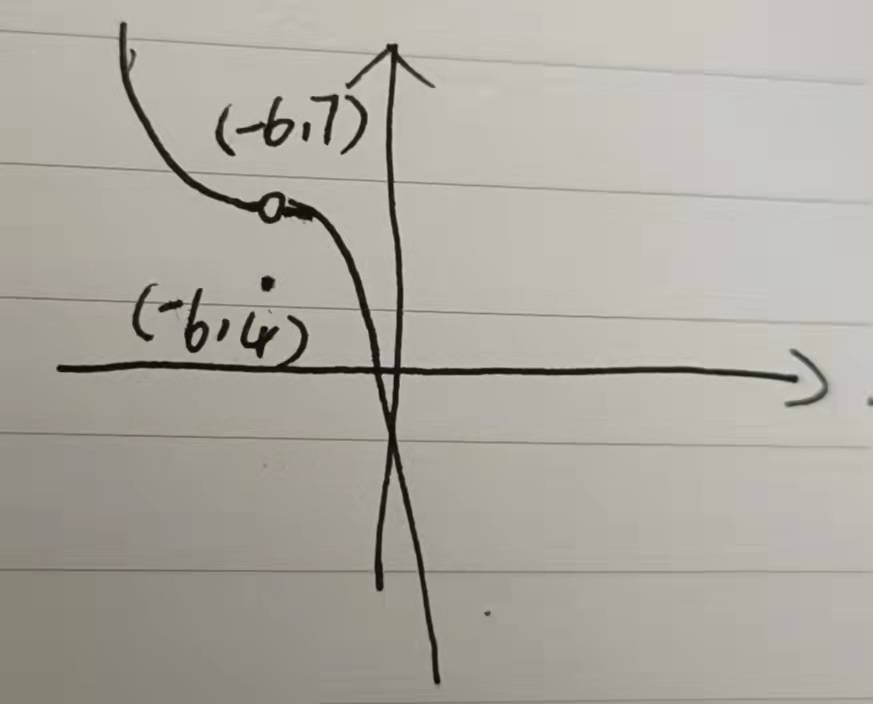### ¿Todavía tienes preguntas de matemáticas?

Pregunte a nuestros tutores expertos
Algebra
PreguntaA function $$f ( x )$$ is said to have a removable discontinuity at $$x = a$$. if both of the following conditions hold:

1. $$f$$ is either not defined or not continuous at $$x = a$$ .

2. $$f ( a )$$ could either be defined or redefined so that the new function is continuous at $$x = a$$ .

show that

has a removable discontinuity at x=-6y by

(a) verifying $$( 1 )$$ in the definition above, and then

(b) verifying $$( 2 )$$ in the definition above by determining a value of $$f ( - 6 )$$ that would make $$f$$ continuous at $$x = - 6 .$$

$$f ( - 6 ) =$$ ? would make $$f$$ continuous at $$x = - 6$$

Now draw a graph of $$f ( x )$$ . It's just a couple of parabolas!